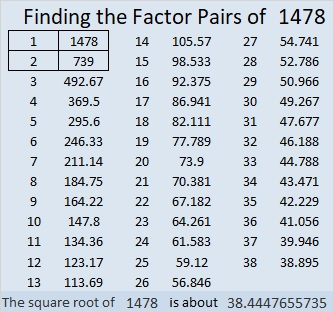# 1478 and Level 1

Contents

### Today’s Puzzle:

If you can divide a few even numbers in half, then you can find all the factors needed to solve this puzzle. Then you can complete the multiplication table that the puzzle makes. Go for it!### Factors of 1478:

• 1478 is a composite number.
• Prime factorization: 1478 = 2 × 739
• 1478 has no exponents greater than 1 in its prime factorization, so √1478 cannot be simplified.
• The exponents in the prime factorization are 1, and 1. Adding one to each exponent and multiplying we get (1 + 1)(1 + 1) = 2 × 2 = 4. Therefore 1478 has exactly 4 factors.
• The factors of 1478 are outlined with their factor pair partners in the graphic below.### A little more about the number 1478:

1478 is not the sum or the difference of two squares, but it is the short leg in a Pythagorean triple:
(1478-546120-546122) calculated from 2(739)(1), 739² – 1², 739² + 1².

This site uses Akismet to reduce spam. Learn how your comment data is processed.